# Calculation Workbooks

## General

A FLOCALC calculation workbook is a collection of FLOCALC calculations which are saved as a single file. The purpose of a workbook is to keep related calculations together and to enable passing data from one calculation to another or to share inputs. If you have Microsoft Excel a more convenient method of passing data from one calculation to another may be to use FloXL the Microsoft Excel interface which is included with FLOCALC.

## Creating a new workbook

To create a FLOCALC calculation workbook click on the Workbook button on the Home ribbon or the New Workbook button on the Workbook ribbon. When the new workbook is created the Workbook button will be selected.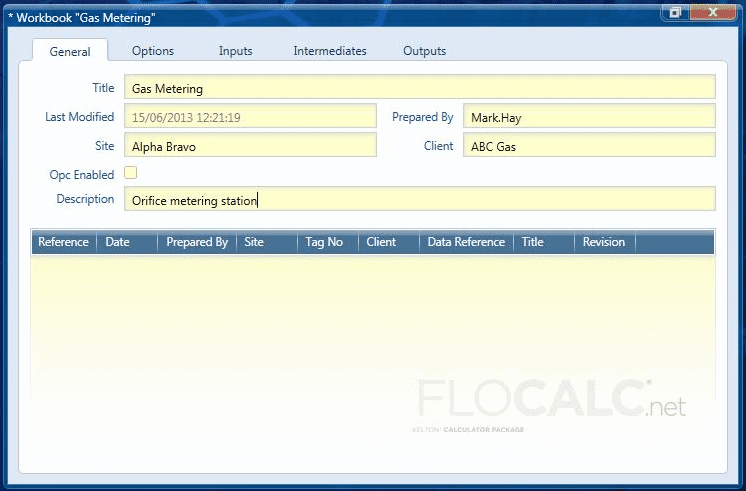Header information can be entered into the Workbook as shown above.

## Adding calculations from the library

To add a new calculation from the library, click the Add from Library button on the Workbook ribbon.

## Adding calculations from a template

To add a new calculation based on a template click the Add from Template button on the Workbook ribbon.

If you wish to import a calculation which has previously been created and saved, open the calculation as using the open button on the Home ribbon.

## Using Workbooks

Once a workbook has been created and calculations have been added they will appear in a list: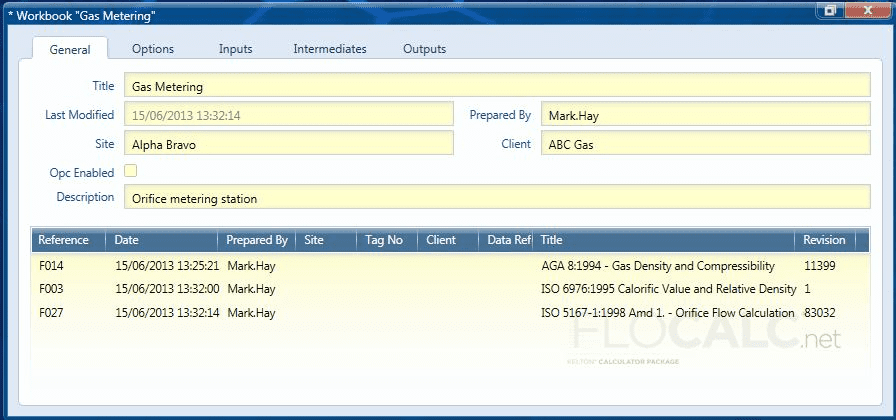Calculations in the workbook can be opened and used in the same way as stand-alone calculations. Alternatively the tabs at the top of the window can be used for setting options, entering inputs and viewing results from any calculation. In this example we are going to create a workbook to calculate the mass and energy flow rate through an orifice meter. The flow rate is calculated in accordance with ISO 5167, the density is calculated using AGA 8 and the calorific value is calculated using ISO 6976. When adding calculations to a workbook the order is significant; calculations can only use values from other calculations if they appear before them in the list.

## Setting Calculation Options

The options tab will let you expand each calculation in the workbook and set the options.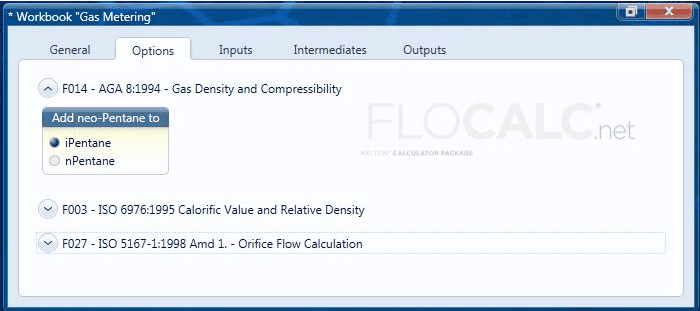## Inputs

The Inputs from for each calculation are grouped and can be expanded by clicking on the arrow to enter the values and select the engineering units.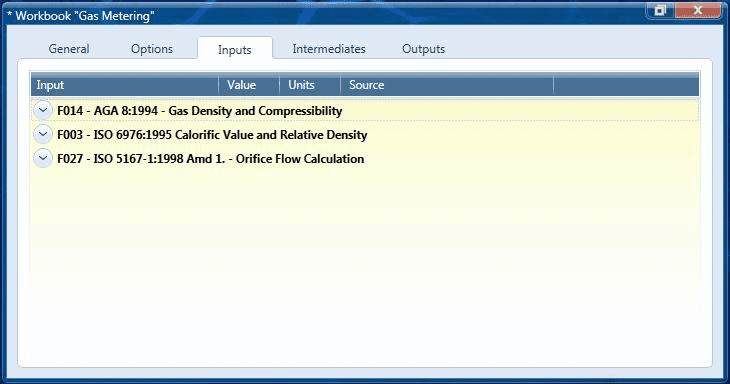On the first calculation in the list the only option available for inputs is User Entered but on subsequent calculations the inputs may be taken from previous calculations in the list. In this example the input composition for F003 ISO 6976 is taken to be the same as the input composition for AGA 8; this will save entering the composition twice.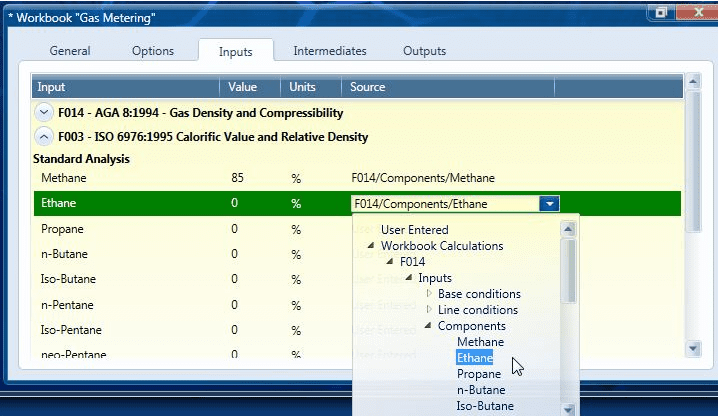The outputs from F014 –AGA 8 and F003 ISO 6976 are fed into F027 – ISO 5167. This example shows the input for the line density required to calculate Flow being linked to the density calculated by AGA 8.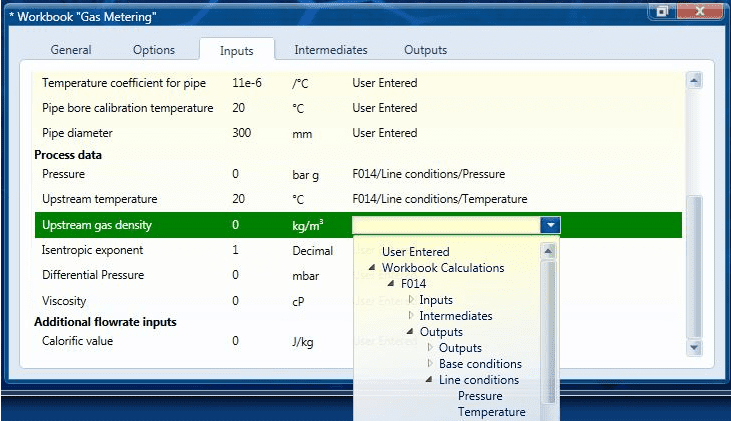Intermediate and output values are displayed for each calculation: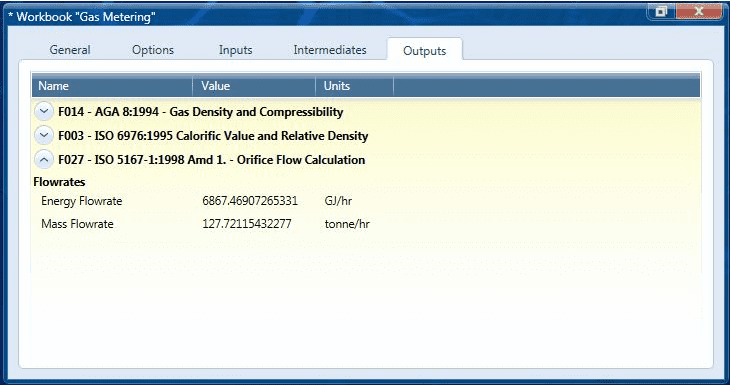Once the links have been set up in a workbook it is recommended that the workbook be saved as a template so that similar calculations can be performed without having to go through the process of setting up links again.

Back